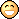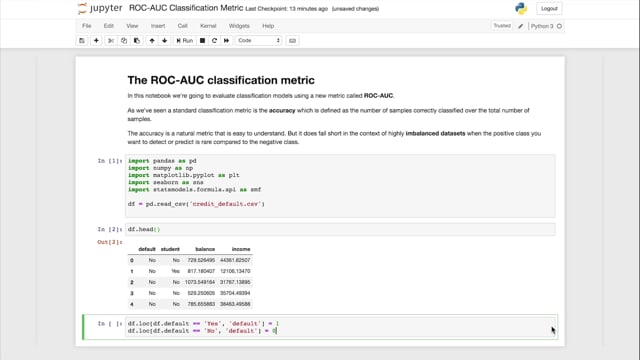Last updated on 5/27/20

## Evaluate Classification ModelsTo measure the predictive power of a linear regression model, use the RMSE metric.

For classification and logistic regression, there are many available metrics, and choosing the right one can be far from obvious. In this last chapter, we will focus on two most useful ones: accuracy and ROC-AUC.

Why these two metrics for classification you ask?

• Accuracy focuses on the amount of correctly classified samples and is simple to understand, but fails when the classes are extremely imbalanced.

• ROC-AUC brings a better understanding of the model classification performance by also taking into account the amount of misclassified samples. It is also robust with respect to imbalanced datasets.

Both metrics can be deduced from the four basic measures: the false and true positives, false and true negatives you saw in the chapter on logistic regression.

In this chapter, you will learn:

• How to define accuracy.

• The ROC curve and the AUC (area under the curve) metric.

We will work with the credit default dataset and the confusion matrix to illustrate accuracy and ROC-AUC.

As its name implies, accuracy is the ration of correctly-classified samples over the total number of samples.

In terms of true positive (TP) and true negatives (TN), the accuracy is defined as:

Let's reload the credit default dataset, fit a logistic regression model, and print the confusion matrix:

import statsmodels.formula.api as smf
import pandas as pd

model = smf.logit('default ~ income + balance + student', data = df)
results = model.fit()
print(results.pred_table())


Recall the confusion matrix:

 Predicted Default Predicted Non-Default Actual Default 286 47 Actual Non-Default 40 460

The accuracy of our model is defined as the number of samples the model classified correctly over the total number of samples.

• Number of samples correctly classified : 460 + 286 = 746.

• Total number of samples : 833.

Our accuracy is 746 / 833 = 90.0%.

Pretty good score, high five!Remember that for each sample, the logit model outputs the probability that the sample belongs to one class . By arbitrarily setting a threshold t=0.5, we conclude that:

• If  , then belongs to the 0 class.

• If  , then belongs to the 1 class.

Setting the threshold to 0.5 to separate the classes is a question of convention. There's no reason why you could not consider a lower or higher threshold. You get different classifications for different thresholds.

For t =0.75, we have and accuracy of 86.91, and the following confusion matrix:

 Predicted Default Predicted Non-Default Actual Default 243 90 Actual Non-Default 19 481

The true and false, positive and negative ratios evolve with the threshold t.

• TPR = TP / Positives = f(t)

• FPR = FP / Positives = g(t)

By plotting the TPR against the FPR while varying the threshold, you obtain the receiver operating characteristic curve, or ROC curve.

The ROC curve illustrates the trade-off between good identification of the truly positive samples, and the misinterpretation of negative samples into the positive class.

The ROC curve for our model is:

We used the scikit-learn library to obtain the true positive rate and false positive rate:

from sklearn.metrics import roc_curve, auc
yhat= results.predict()
false_positive_rate, true_positive_rate, thresholds = roc_curve(df['default'], yhat)


#### Interpreting the ROC Curve

We have a nice curve and a straight line (red). How do they play with each other?

The straight red line is the reference. It corresponds to a random model where we simply flip a coin to classify the samples in either class. The further away the ROC curve is from that straight line, the better the model.

• As the curve gets closer to the upper-left corner of the plot, the TPR becomes larger than the FPR as we increase the threshold. We get more correctly identified samples than incorrectly identified ones. Our model works!

• The sooner the curve moves to the upper-left corner, the better. It means that our model discriminates well between the classes.

#### The AUC

Graphic interpretation is great, but having a single number would be better for model selection.

The area under the curve (AUC) is such a metric:

• The AUC goes from 0.5 to 1.

• The larger the better.

Some values:

• AUC of 0.9 and above means jackpot! The model is excellent.

• Any AUC > 0.7, ..., 0.75 is satisfactory.

• AUC between 0.5 and 0.6/0.7 indicates a poor model.

• An AUC of 0.5 is a random coin-flipping useless model.

Of course, these numbers are all indicative and cannot be blindly applied to all cases. For some datasets, painfully reaching 0.68 AUC will be grounds for celebration, while 0.84 might indicate an urgent need to get back to work on your models.

You may remember that in the credit default dataset we used in a previous chapter is not the original one.

The original dataset, which is available here, has a large majority of negative samples.

• No: 9667

• Yes: 333

For a total of 10000 samples.

This is a good example of an imbalanced dataset where the interesting class you want to detect or predict is far less frequent than the other less interesting class.

Examples of imbalanced datasets include:

• Customer conversion. How many people on a website end up converting or clicking on a banner or link?

• A good example of this is the caravan dataset that holds information on consumers buying an insurance policy for their caravan. 348 yes, for 5474 no.

• Anomaly detection: intrusion detection, counterfeit note identification.

Consider a model that always predicts that people will not default on their credit. It's not even a logistic regression model; simply a constant value of 0.
The confusion matrix for that ultra simple model is:

 TN: 9667 FN: 333 FP:0 TP:0

This gives an accuracy score of:

Wow! This score is much better than the accuracy score (90.0%) we have with our subsamples dataset logit model! Of course, this model is not relevant at all, since we want to predict the minority class (default) not the majority one (non default).

This absurd accuracy performance of the most simple model on an imbalanced dataset is called the accuracy paradox. It's a good reason why we need another classification metric such as the ROC-AUC.For that, we turn to the scikit-learn library, which includes the right methods to calculate the AUC or plot the ROC curve using predicted probabilities and true values as input.

The roc_auc_score method will output the AUC score:

from sklearn.metrics import roc_auc_score
score = roc_auc_score(y, yhat_proba)


The roc_curve method will output the false positive and true positive ratios.

from sklearn.metrics import roc_curve
fpr, tpr, thresholds = metrics.roc_curve(y, yhat_proba)


Plotting the ROC curve boils down to plotting the TPR vs. the FPR.

#### Accuracy vs. AUC - the Duel

Let's compare how AUC handles itself when faced with a highly imbalanced dataset:

model = smf.logit('default ~ income + balance + student', data = df)


Built on the full imbalanced dataset  df = pd.read_csv('credit_default.csv'), and the sampled balanced dataset  df = pd.read_csv('credit_default_sampled.csv').

We end up with:

• Credit_default.csv AUC: 0.95.

• Credit_default_sampled.csv AUC: 0.96.

The AUC handles itself well in presence of an imbalanced dataset.

In this last chapter, you learned about the following items:

• Defining multiple metrics based on the true/false and positive/negative ratios.

• Interpreting the ROC curve and calculating the AUC score.

• The accuracy paradox when dealing with imbalanced datasets.

Since the dawn of time, humanity has probably tried to define relevant metrics for classification problems. These problems arise in many different areas: from medical to marketing, security to finance, and so forth across different disciplines.

This has lead to a multitude of classification metrics tailored to specific contexts. To add to the confusion, the same metrics can have different names.

For instance, the TPR is also called sensitivity, recall, or hit rate. Listing all the classification metrics with their different definitions and names would not be very useful. It's worth mentioning that scikit-learn offers a simple classification_report method that lists the most important classification metrics.

from sklearn.metrics import classification_report
print(confusion_matrix(df['default'], predicted_class))


Outputs for the balanced reduced dataset:

 precision recall f1-score support 0 0.91 0.92 0.91 500 1 0.88 0.86 0.87 333

For the entire dataset, we have:

 precision recall f1-score support 0 0.98 1 0.99 9667 1 0.74 0.32 0.45 333

Where:

• Precision = TP / TP + FP

• Recall = TPR = TP / (TP + FN) = TP / All positives

• f1-score = is the harmonic mean of precision and sensitivity defined as:

Comparing the classification report for the two models, we see that recall and f1-score are particularly sensitive to the imbalance aspect of the dataset.